DEPY MAKRI

•Copy of Will It Hit The Hoop?

Activity

DEPY MAKRI

•Scientific Notation

Activity

DEPY MAKRI

•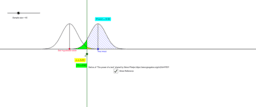The Power of a Test

Activity

DEPY MAKRI

•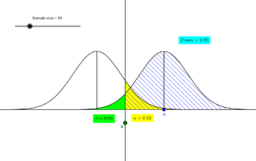Copy of The Power of a Test made by Steve Phelps

Activity

DEPY MAKRI

•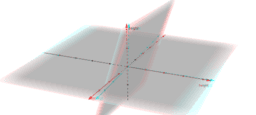3-d regression analysis output

Activity

DEPY MAKRI

•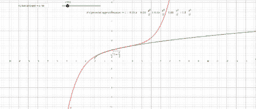taylor series expansion

Activity

DEPY MAKRI

•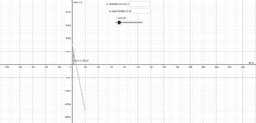area under velocity time graph

Activity

DEPY MAKRI

•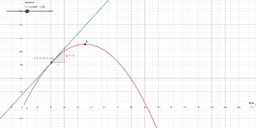THE GRADIENT OF THE POSITION TIME GRAPH

Activity

DEPY MAKRI

•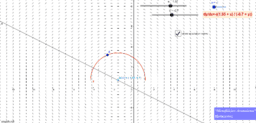an ordinary differential equation with two parameters-investigation

Activity

DEPY MAKRI

•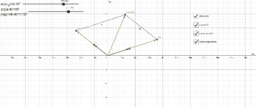multiplying complex numbers using their polar form intro

Activity

DEPY MAKRI

•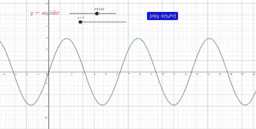Play with sine

Activity

DEPY MAKRI

•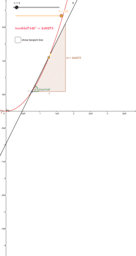mei-a level Y1

Book

DEPY MAKRI

•Activity

DEPY MAKRI

•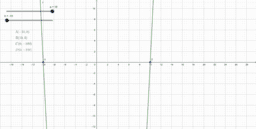page 33 technology activity

Activity

DEPY MAKRI

•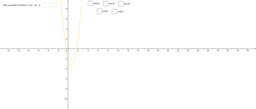Activity

DEPY MAKRI

•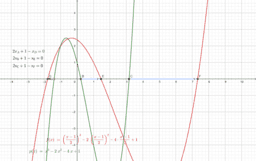equations with roots related by a linear transformation

Activity

DEPY MAKRI

•Loci on the Argand Plane

Book

DEPY MAKRI

•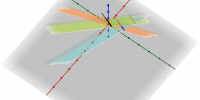3 non parallel planes with parallel lines of intersection

Activity

DEPY MAKRI

•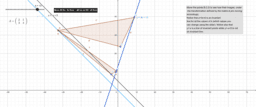invariant lines and line of invariant points

Activity

DEPY MAKRI

•question 10 i,ii,iii

Activity

DEPY MAKRI

•Brief and analytic guidelines for visualising complex loci using Geogebra part 1

Activity

DEPY MAKRI

•Brief and analytic guidelines for visualising the loci 1st part

Activity

DEPY MAKRI

•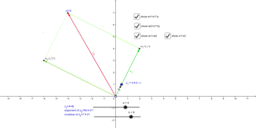Multiplying complex numbers using their polar form:geometrical proof

Activity

DEPY MAKRI

•Activity

DEPY MAKRI